Next: Optimal Combination Up: Statistical Background Previous: Parameter Estimation

## Covariance Estimation

The concept of error covariance is very important in statistics as it allows us to model linear correlations between parameters. For locally linear fit functions f we can approximate the variation in a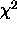metric about the minimum value as a quadratic. We will examine the two dimensional case first, for example: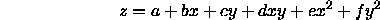This can be written as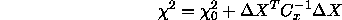where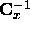is defined as the inverse covariance matrix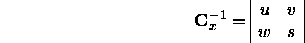Comparing with the above quadratic equation we get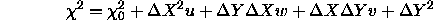where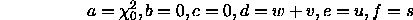Notice that the b and c coefficients are zero as required if theis at the minimum. In the general case we need a method for determining the covariance matrix for model fits with an arbitrary number of parameters. Starting from thedefinition using the same notation as previously.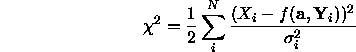We can compute the first and second order derivatives as follows: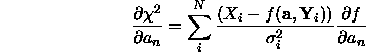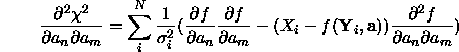The second term in this equation is expected to be negligible compared to the first and with an expected value of zero if the model is a good fit. Thus the cross derivatives can be approximated to a good accuracy by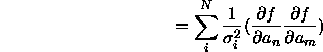The following quantities are often defined.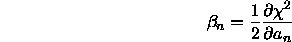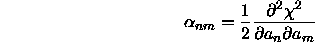As these derivatives must correspond to the first coefficients in a polynomial (Taylor) expansion of thefunction then,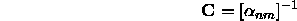And the expected change infor a small change in model parameters can be written as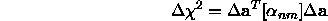Bob Fisher
Fri Mar 28 14:12:50 GMT 1997